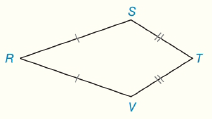Chapter 4.CT, Problem 17CT### Elementary Geometry for College St...

6th Edition
Daniel C. Alexander + 1 other
ISBN: 9781285195698

#### Solutions

Chapter
Section### Elementary Geometry for College St...

6th Edition
Daniel C. Alexander + 1 other
ISBN: 9781285195698
Textbook Problem
17 views

# In Kite RSTV, R S = 2 x − 4 , S T = x − 1 , T V = y − 3     and  R V = y . Find the perimeter of RSTV.To determine

To Find:

The perimeter of kite.

Explanation

Given:

In Kite RSTV, RS=2x4,ST=x1,TV=y3 and RV=y. RSTV.

Calculation:

From given figure it is clear that RSRV and STTV.

Consider RSRV then,

2x4=y2xy=4(1)

Now consider STTV then,

x1=y3xy=13xy=2(2)

By subtracting equation (1) from (2) we have,

2xy=4xy=2_x+0y=6

Thus, x=6.

Substitute for x in (2) we have,

### Still sussing out bartleby?

Check out a sample textbook solution.

See a sample solution

#### The Solution to Your Study Problems

Bartleby provides explanations to thousands of textbook problems written by our experts, many with advanced degrees!

Get Started

#### Find the minimum cost C (in dollars), given that 5(C 25) 1.75 + 2.5C

Applied Calculus for the Managerial, Life, and Social Sciences: A Brief Approach

#### Expand each expression in Exercises 122. (x+1x)2

Finite Mathematics and Applied Calculus (MindTap Course List)

#### The quadratic approximation for at a = 1 is:

Study Guide for Stewart's Multivariable Calculus, 8th